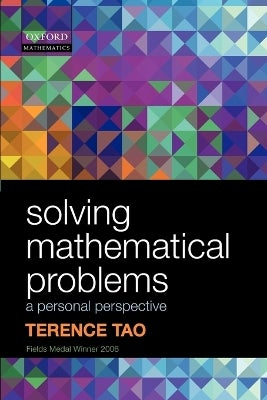•Free Click & Collect
•Free delivery on orders over \$49.99
•on orders of \$50 or more# Solving Mathematical Problems

###### A Personal Perspective

By Terence Tao

Paperback

\$60.60

Or 4 payments of \$15.15 with

Delivered in 10 - 14 days
Available for Click and Collect

#### Toggle filter item Description

From Terence Tao, Fields Medal Winner 2006, Solving Mathematical Problems is an engaging and clearly presented text that leads the reader through the various tactics involved in solving mathematical problems at the Mathematical Olympiad level. Covering number theory, algebra, analysis, Euclidean geometry and analytic geometry, Solving Mathematical Problems includes numerous exercises and model solutions throughout. Assuming only a basic level of mathematics, the text is ideal for students of 14 years and above in pure mathematics.

Product code: 9780199205608

ISBN 9780199205608 H234xW155xS8 128 Oxford University Press

#### Toggle filter item Synopsis

Authored by a leading name in mathematics, this engaging and clearly presented text leads the reader through the tactics involved in solving mathematical problems at the Mathematical Olympiad level. With numerous exercises and assuming only basic mathematics, this text is ideal for students of 14 years and above in pure mathematics.